# 6.2 Graphs of exponential functions  (Page 6/6)

 Page 6 / 6

## Verbal

What role does the horizontal asymptote of an exponential function play in telling us about the end behavior of the graph?

An asymptote is a line that the graph of a function approaches, as $\text{\hspace{0.17em}}x\text{\hspace{0.17em}}$ either increases or decreases without bound. The horizontal asymptote of an exponential function tells us the limit of the function’s values as the independent variable gets either extremely large or extremely small.

What is the advantage of knowing how to recognize transformations of the graph of a parent function algebraically?

## Algebraic

The graph of $\text{\hspace{0.17em}}f\left(x\right)={3}^{x}\text{\hspace{0.17em}}$ is reflected about the y -axis and stretched vertically by a factor of $\text{\hspace{0.17em}}4.\text{\hspace{0.17em}}$ What is the equation of the new function, $\text{\hspace{0.17em}}g\left(x\right)?\text{\hspace{0.17em}}$ State its y -intercept, domain, and range.

$g\left(x\right)=4{\left(3\right)}^{-x};\text{\hspace{0.17em}}$ y -intercept: $\text{\hspace{0.17em}}\left(0,4\right);\text{\hspace{0.17em}}$ Domain: all real numbers; Range: all real numbers greater than $\text{\hspace{0.17em}}0.$

The graph of $\text{\hspace{0.17em}}f\left(x\right)={\left(\frac{1}{2}\right)}^{-x}\text{\hspace{0.17em}}$ is reflected about the y -axis and compressed vertically by a factor of $\text{\hspace{0.17em}}\frac{1}{5}.\text{\hspace{0.17em}}$ What is the equation of the new function, $\text{\hspace{0.17em}}g\left(x\right)?\text{\hspace{0.17em}}$ State its y -intercept, domain, and range.

The graph of $\text{\hspace{0.17em}}f\left(x\right)={10}^{x}\text{\hspace{0.17em}}$ is reflected about the x -axis and shifted upward $\text{\hspace{0.17em}}7\text{\hspace{0.17em}}$ units. What is the equation of the new function, $\text{\hspace{0.17em}}g\left(x\right)?\text{\hspace{0.17em}}$ State its y -intercept, domain, and range.

$g\left(x\right)=-{10}^{x}+7;\text{\hspace{0.17em}}$ y -intercept: $\text{\hspace{0.17em}}\left(0,6\right);\text{\hspace{0.17em}}$ Domain: all real numbers; Range: all real numbers less than $\text{\hspace{0.17em}}7.$

The graph of $\text{\hspace{0.17em}}f\left(x\right)={\left(1.68\right)}^{x}\text{\hspace{0.17em}}$ is shifted right $\text{\hspace{0.17em}}3\text{\hspace{0.17em}}$ units, stretched vertically by a factor of $\text{\hspace{0.17em}}2,$ reflected about the x -axis, and then shifted downward $\text{\hspace{0.17em}}3\text{\hspace{0.17em}}$ units. What is the equation of the new function, $\text{\hspace{0.17em}}g\left(x\right)?\text{\hspace{0.17em}}$ State its y -intercept (to the nearest thousandth), domain, and range.

The graph of $\text{\hspace{0.17em}}f\left(x\right)=2{\left(\frac{1}{4}\right)}^{x-20}$ is shifted left $\text{\hspace{0.17em}}2\text{\hspace{0.17em}}$ units, stretched vertically by a factor of $\text{\hspace{0.17em}}4,$ reflected about the x -axis, and then shifted downward $\text{\hspace{0.17em}}4\text{\hspace{0.17em}}$ units. What is the equation of the new function, $\text{\hspace{0.17em}}g\left(x\right)?\text{\hspace{0.17em}}$ State its y -intercept, domain, and range.

$g\left(x\right)=2{\left(\frac{1}{4}\right)}^{x};\text{\hspace{0.17em}}$ y -intercept: Domain: all real numbers; Range: all real numbers greater than $\text{\hspace{0.17em}}0.$

## Graphical

For the following exercises, graph the function and its reflection about the y -axis on the same axes, and give the y -intercept.

$f\left(x\right)=3{\left(\frac{1}{2}\right)}^{x}$

$g\left(x\right)=-2{\left(0.25\right)}^{x}$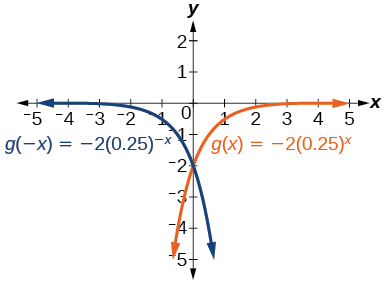y -intercept: $\text{\hspace{0.17em}}\left(0,-2\right)$

$h\left(x\right)=6{\left(1.75\right)}^{-x}$

For the following exercises, graph each set of functions on the same axes.

$f\left(x\right)=3{\left(\frac{1}{4}\right)}^{x},$ $g\left(x\right)=3{\left(2\right)}^{x},$ and $\text{\hspace{0.17em}}h\left(x\right)=3{\left(4\right)}^{x}$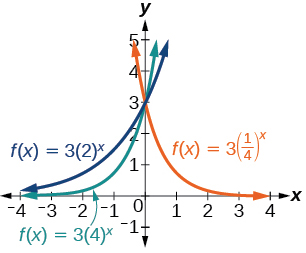$f\left(x\right)=\frac{1}{4}{\left(3\right)}^{x},$ $g\left(x\right)=2{\left(3\right)}^{x},$ and $\text{\hspace{0.17em}}h\left(x\right)=4{\left(3\right)}^{x}$

For the following exercises, match each function with one of the graphs in [link] .

$f\left(x\right)=2{\left(0.69\right)}^{x}$

B

$f\left(x\right)=2{\left(1.28\right)}^{x}$

$f\left(x\right)=2{\left(0.81\right)}^{x}$

A

$f\left(x\right)=4{\left(1.28\right)}^{x}$

$f\left(x\right)=2{\left(1.59\right)}^{x}$

E

$f\left(x\right)=4{\left(0.69\right)}^{x}$

For the following exercises, use the graphs shown in [link] . All have the form $\text{\hspace{0.17em}}f\left(x\right)=a{b}^{x}.$

Which graph has the largest value for $\text{\hspace{0.17em}}b?$

D

Which graph has the smallest value for $\text{\hspace{0.17em}}b?$

Which graph has the largest value for $\text{\hspace{0.17em}}a?$

C

Which graph has the smallest value for $\text{\hspace{0.17em}}a?$

For the following exercises, graph the function and its reflection about the x -axis on the same axes.

$f\left(x\right)=\frac{1}{2}{\left(4\right)}^{x}$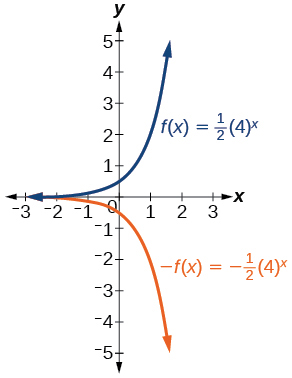$f\left(x\right)=3{\left(0.75\right)}^{x}-1$

$f\left(x\right)=-4{\left(2\right)}^{x}+2$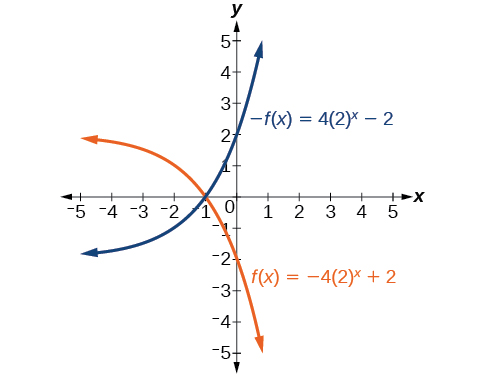For the following exercises, graph the transformation of $\text{\hspace{0.17em}}f\left(x\right)={2}^{x}.\text{\hspace{0.17em}}$ Give the horizontal asymptote, the domain, and the range.

$f\left(x\right)={2}^{-x}$

$h\left(x\right)={2}^{x}+3$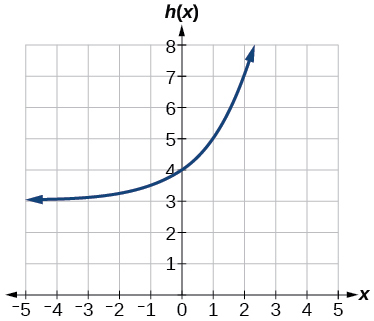Horizontal asymptote: $\text{\hspace{0.17em}}h\left(x\right)=3;$ Domain: all real numbers; Range: all real numbers strictly greater than $\text{\hspace{0.17em}}3.$

$f\left(x\right)={2}^{x-2}$

For the following exercises, describe the end behavior of the graphs of the functions.

$f\left(x\right)=-5{\left(4\right)}^{x}-1$

As $x\to \infty$ , $f\left(x\right)\to -\infty$ ;
As $x\to -\infty$ , $f\left(x\right)\to -1$

$f\left(x\right)=3{\left(\frac{1}{2}\right)}^{x}-2$

$f\left(x\right)=3{\left(4\right)}^{-x}+2$

As $x\to \infty$ , $f\left(x\right)\to 2$ ;
As $x\to -\infty$ , $f\left(x\right)\to \infty$

For the following exercises, start with the graph of $\text{\hspace{0.17em}}f\left(x\right)={4}^{x}.\text{\hspace{0.17em}}$ Then write a function that results from the given transformation.

Shift $f\left(x\right)$ 4 units upward

Shift $\text{\hspace{0.17em}}f\left(x\right)\text{\hspace{0.17em}}$ 3 units downward

$f\left(x\right)={4}^{x}-3$

Shift $\text{\hspace{0.17em}}f\left(x\right)\text{\hspace{0.17em}}$ 2 units left

Shift $\text{\hspace{0.17em}}f\left(x\right)\text{\hspace{0.17em}}$ 5 units right

$f\left(x\right)={4}^{x-5}$

Reflect $\text{\hspace{0.17em}}f\left(x\right)\text{\hspace{0.17em}}$ about the x -axis

Reflect $\text{\hspace{0.17em}}f\left(x\right)\text{\hspace{0.17em}}$ about the y -axis

$f\left(x\right)={4}^{-x}$

For the following exercises, each graph is a transformation of $\text{\hspace{0.17em}}y={2}^{x}.\text{\hspace{0.17em}}$ Write an equation describing the transformation.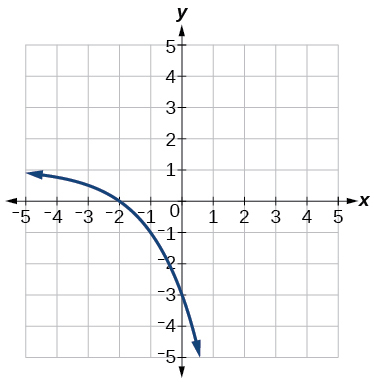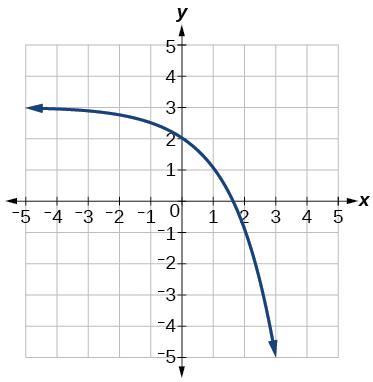$y=-{2}^{x}+3$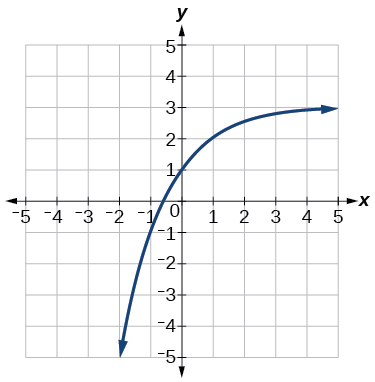For the following exercises, find an exponential equation for the graph.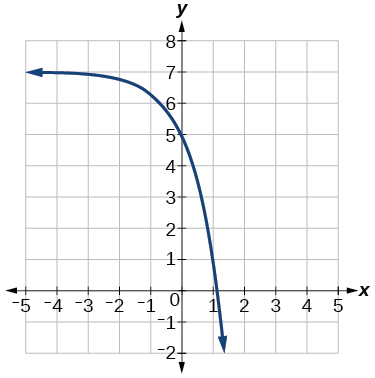$y=-2{\left(3\right)}^{x}+7$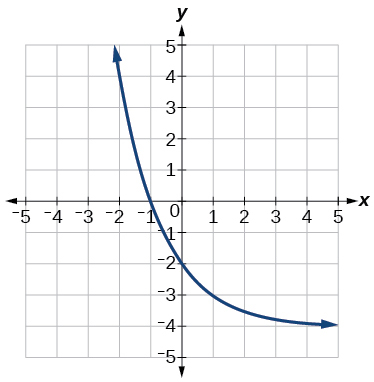## Numeric

For the following exercises, evaluate the exponential functions for the indicated value of $\text{\hspace{0.17em}}x.$

$g\left(x\right)=\frac{1}{3}{\left(7\right)}^{x-2}\text{\hspace{0.17em}}$ for $\text{\hspace{0.17em}}g\left(6\right).$

$g\left(6\right)=800+\frac{1}{3}\approx 800.3333$

$f\left(x\right)=4{\left(2\right)}^{x-1}-2\text{\hspace{0.17em}}$ for $\text{\hspace{0.17em}}f\left(5\right).$

$h\left(x\right)=-\frac{1}{2}{\left(\frac{1}{2}\right)}^{x}+6\text{\hspace{0.17em}}$ for $\text{\hspace{0.17em}}h\left(-7\right).$

$h\left(-7\right)=-58$

## Technology

For the following exercises, use a graphing calculator to approximate the solutions of the equation. Round to the nearest thousandth. $\text{\hspace{0.17em}}f\left(x\right)=a{b}^{x}+d.$

$-50=-{\left(\frac{1}{2}\right)}^{-x}$

$116=\frac{1}{4}{\left(\frac{1}{8}\right)}^{x}$

$x\approx -2.953$

$12=2{\left(3\right)}^{x}+1$

$5=3{\left(\frac{1}{2}\right)}^{x-1}-2$

$x\approx -0.222$

$-30=-4{\left(2\right)}^{x+2}+2$

## Extensions

Explore and discuss the graphs of $\text{\hspace{0.17em}}F\left(x\right)={\left(b\right)}^{x}\text{\hspace{0.17em}}$ and $\text{\hspace{0.17em}}G\left(x\right)={\left(\frac{1}{b}\right)}^{x}.\text{\hspace{0.17em}}$ Then make a conjecture about the relationship between the graphs of the functions $\text{\hspace{0.17em}}{b}^{x}\text{\hspace{0.17em}}$ and $\text{\hspace{0.17em}}{\left(\frac{1}{b}\right)}^{x}\text{\hspace{0.17em}}$ for any real number $\text{\hspace{0.17em}}b>0.$

The graph of $\text{\hspace{0.17em}}G\left(x\right)={\left(\frac{1}{b}\right)}^{x}\text{\hspace{0.17em}}$ is the refelction about the y -axis of the graph of $\text{\hspace{0.17em}}F\left(x\right)={b}^{x};\text{\hspace{0.17em}}$ For any real number $\text{\hspace{0.17em}}b>0\text{\hspace{0.17em}}$ and function $\text{\hspace{0.17em}}f\left(x\right)={b}^{x},$ the graph of $\text{\hspace{0.17em}}{\left(\frac{1}{b}\right)}^{x}\text{\hspace{0.17em}}$ is the the reflection about the y -axis, $\text{\hspace{0.17em}}F\left(-x\right).$

Prove the conjecture made in the previous exercise.

Explore and discuss the graphs of $\text{\hspace{0.17em}}f\left(x\right)={4}^{x},$ $\text{\hspace{0.17em}}g\left(x\right)={4}^{x-2},$ and $\text{\hspace{0.17em}}h\left(x\right)=\left(\frac{1}{16}\right){4}^{x}.\text{\hspace{0.17em}}$ Then make a conjecture about the relationship between the graphs of the functions $\text{\hspace{0.17em}}{b}^{x}\text{\hspace{0.17em}}$ and $\text{\hspace{0.17em}}\left(\frac{1}{{b}^{n}}\right){b}^{x}\text{\hspace{0.17em}}$ for any real number n and real number $\text{\hspace{0.17em}}b>0.$

The graphs of $\text{\hspace{0.17em}}g\left(x\right)\text{\hspace{0.17em}}$ and $\text{\hspace{0.17em}}h\left(x\right)\text{\hspace{0.17em}}$ are the same and are a horizontal shift to the right of the graph of $\text{\hspace{0.17em}}f\left(x\right);\text{\hspace{0.17em}}$ For any real number n , real number $\text{\hspace{0.17em}}b>0,$ and function $\text{\hspace{0.17em}}f\left(x\right)={b}^{x},$ the graph of $\text{\hspace{0.17em}}\left(\frac{1}{{b}^{n}}\right){b}^{x}\text{\hspace{0.17em}}$ is the horizontal shift $\text{\hspace{0.17em}}f\left(x-n\right).$

Prove the conjecture made in the previous exercise.

#### Questions & Answers

factoring polynomial
find general solution of the Tanx=-1/root3,secx=2/root3
find general solution of the following equation
Nani
the value of 2 sin square 60 Cos 60
0.75
Lynne
0.75
Inkoom
when can I use sin, cos tan in a giving question
depending on the question
Nicholas
I am a carpenter and I have to cut and assemble a conventional roof line for a new home. The dimensions are: width 30'6" length 40'6". I want a 6 and 12 pitch. The roof is a full hip construction. Give me the L,W and height of rafters for the hip, hip jacks also the length of common jacks.
John
I want to learn the calculations
where can I get indices
I need matrices
Nasasira
hi
Raihany
Hi
Solomon
need help
Raihany
maybe provide us videos
Nasasira
Raihany
Hello
Cromwell
a
Amie
What do you mean by a
Cromwell
nothing. I accidentally press it
Amie
you guys know any app with matrices?
Khay
Ok
Cromwell
Solve the x? x=18+(24-3)=72
x-39=72 x=111
Suraj
Solve the formula for the indicated variable P=b+4a+2c, for b
Need help with this question please
b=-4ac-2c+P
Denisse
b=p-4a-2c
Suddhen
b= p - 4a - 2c
Snr
p=2(2a+C)+b
Suraj
b=p-2(2a+c)
Tapiwa
P=4a+b+2C
COLEMAN
b=P-4a-2c
COLEMAN
like Deadra, show me the step by step order of operation to alive for b
John
A laser rangefinder is locked on a comet approaching Earth. The distance g(x), in kilometers, of the comet after x days, for x in the interval 0 to 30 days, is given by g(x)=250,000csc(π30x). Graph g(x) on the interval [0, 35]. Evaluate g(5)  and interpret the information. What is the minimum distance between the comet and Earth? When does this occur? To which constant in the equation does this correspond? Find and discuss the meaning of any vertical asymptotes.
The sequence is {1,-1,1-1.....} has
circular region of radious
how can we solve this problem
Sin(A+B) = sinBcosA+cosBsinA
Prove it
Eseka
Eseka
hi
Joel
yah
immy
June needs 45 gallons of punch. 2 different coolers. Bigger cooler is 5 times as large as smaller cooler. How many gallons in each cooler?
7.5 and 37.5
Nando
how would this look as an equation?
Hayden
5x+x=45
KhayByByByBy